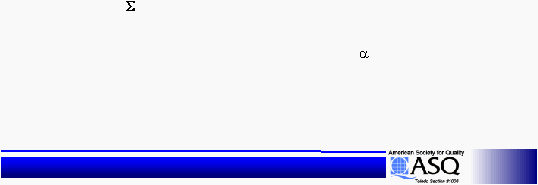< > unit5ReadOnly - page 12 of 13 Six Sigma Six Sigma Training - 6 SigmaStatistical Design of Experiments
Statistical Design of Experiments
Reference
Reference
Process Average:
Process Average:
Gives a prediction of the response
Gives a prediction of the response
variable using the significant input factors at their
variable using the significant input factors at their
desired levels.
desired levels.
T-bar
T-bar
= Average of all of the test results
= Average of all of the test results
Mean =  (
Mean (
(
(
Significant Input Factor Level
Significant Input Factor Level
Average
Average
i
i
) -
) &#45;
-
(n-1)*T-bar
(n&#45;1)*T&#45;bar
-1)*T&#38;#45;bar
1)*T&#38;#38;#45;bar
-bar
bar
Confidence Interval
Confidence Interval
= Square Root (F
= Square Root (F
*
*
V
V
err
err
/
/
n
n
eff
eff
)
)
n
n
eff
eff
= N/1 + [total degrees of freedom associated with
= N/1 + [total degrees of freedom associated with
factors used in process average estimate]
factors used in process average estimate]
n
i=1
,1,df
err